# Nets of 3D Shapes

GCSELevel 1-3Cambridge iGCSEWJEC

## Nets of 3D Shapes

Nets of 3D shapes are what the shape would look like if it was opened and laid out flat. You may need to identify or draw nets of different 3D shapes.

## Nets Introduction

The net of a 3D shape can be folded into the 3D shape.

For example, this is a net of a cube:The net above can be folded to create the cube, as follows: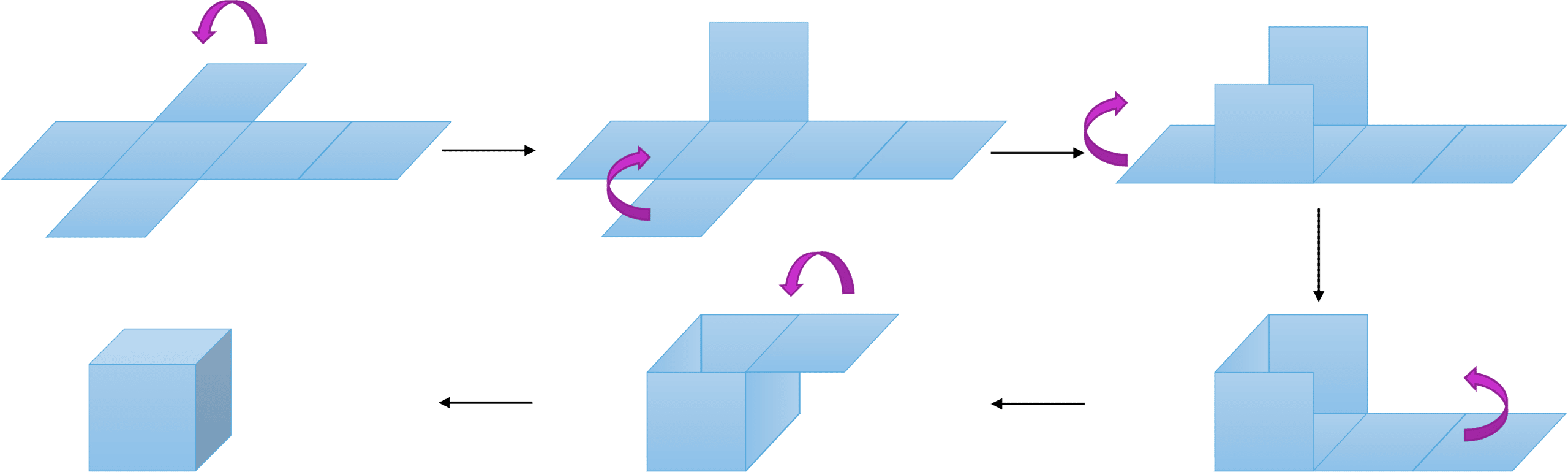Level 1-3GCSEWJECCambridge iGCSE

## Nets Overview

Below are examples of some nets of some 3D shapes. It is important to note, however, that some of the shapes may have more than one possible net (for example, a cube has $11$ ways of formatting a net, all consisting of $6$ squares in a different arrangement.)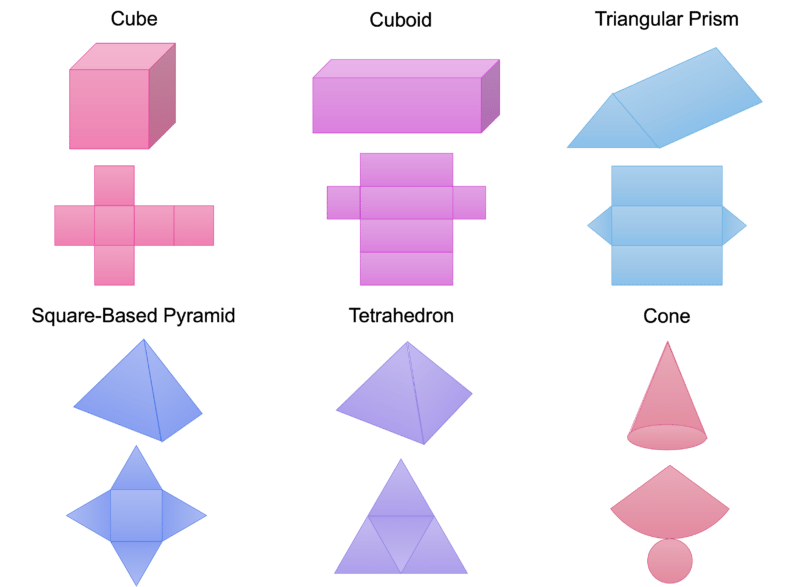Level 1-3GCSEWJECCambridge iGCSE

## Example 1: Identifying Nets

Identify which of the following are nets of a cube.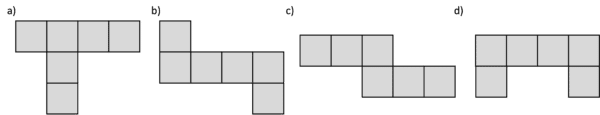[4 marks]

Let’s go through each net and visualise or draw whether this could fold into a 3D cube.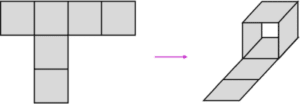This cannot be folded into a cube as there would be no back to the cube.

Now for b)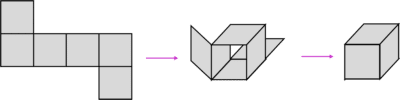This can be made into a cube.

c)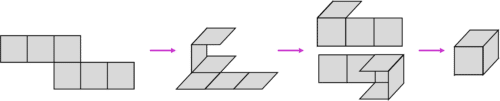This can also be made into a cube.

And finally, d)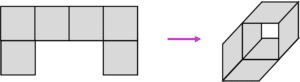This cannot be folded into a cube as there would be no back for the cube.

Therefore, b and c are nets of a cube.

Level 1-3GCSEWJECCambridge iGCSE

## Example 2: Drawing a Net

Draw the net of a cylinder.

[3 marks]

To draw a net, start by considering which faces the shape has:

A cylinder has two circular faces and one curved rectangular face.

Then consider the faces’ arrangement:

The circles are at either side of the rectangle.

And finally, draw the net: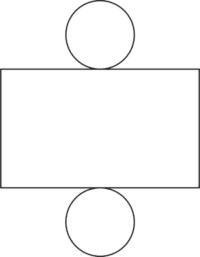Level 1-3GCSEWJECCambridge iGCSE

## Nets of 3D Shapes Example Questions

The net will look like this: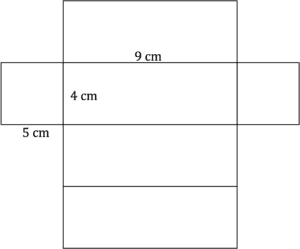b) is the only option that can be correctly folded into a square-based pyramid.

By looking at the options, we can identify that c) is definitely not correct:

c) cannot be correct as the width of the rectangle should be the same as the circumference of the circle, which is not $8\text{ cm}$, as this is the diameter, and the circumference is always longer than the diameter.

To work out whether a) and b) is correct, the circumference of the circle needs to be calculated:

Circumference $= \pi d$

$= 8\pi$ $=25.1...$

Therefore, a) is the correct net.

## You May Also Like...### MME Learning Portal

Online exams, practice questions and revision videos for every GCSE level 9-1 topic! No fees, no trial period, just totally free access to the UK’s best GCSE maths revision platform.

£0.00